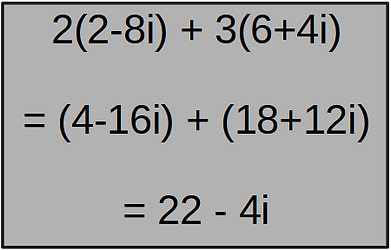top of page# Basics

Working with complex numbers can be quite easy once you learn the basic rules, as they tend to seem harder to work with than how they actually are.

This page will go over basic maths operations for complex numbers which includes :

• Subtraction

• Multiplication

• Division

Following the rules of Complex Number Standard Form (a+bi), addition works by adding like terms. For example, you would sum the Real terms for the new 'a' part and sum the Imaginary terms for the new 'b' part.

Addition of complex numbers can also be achieved, or represented, by using a Complex Plane. This method will be explained in later pages, where we will introduce vectors in cartesian form, and there addition through graphs and other methods.

Like in algebra, multipliers outside of brackets also work with complex numbers. First, distribute the multiplier with the contents of the brackets, then expand and combine like terms as before.

Subtraction of complex numbers

The method of subtraction for complex numbers is essentially what you would expect. You begin as before, distributing any multipliers with the contents of the brackets, then expand the brackets and subtract like terms to get the result.

Simple multiplicationbottom of page